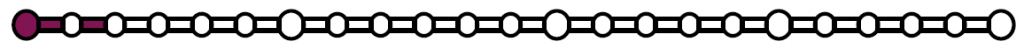FOR_2: Define expected outputIdentify a system to be forecasted and identify what results will be required by the decision makers. Also identify how the decision makers will use the results from the forecast.

Method Method = FORMAT methodology

After the question “Why do we need learn about the future”, now it is time to clarify the “What” do we need to know about the future? It is required to specify the main objectives of the project from the perspective of what is desired to learn about the future, and what we would like to know about the future in order to enhance strategic planning and decision making.

Instructions

1. Answer the following question (to be answered by the working team):
• What do we need to know about the future?
• This question has to have the system to be forecasted (STFSTF = System to be forecasted ) as the central component.
1. During the first working session, clarify and formulate the decision criteria for the STFSTF = System to be forecasted .
2. These questions need to be answered in the above mentioned session:
• What is expected by users and beneficiaries as a result of the forecast?
• What question should be answered for decision makers?
• What is the necessary level of detail?
• How do the results need to be presented? (e.g. graphs, charts, report)
• How will these results be used by users and beneficiaries?
• Will the results of the forecast be used for other purposes? Which ones?
• What is the approximate time span when a result may be used?
• Where are the results going to be useful?
• Who is going to use the results of the forecast?

Tips

➔    Keep in mind that the next step will require you to take a decision on the following question: Can we get the required results without forecast, i.e. by solving a well-identified problem? (Yes/No).

For rules to define function of a system see:

Kucharavy, D.  (2008), “Course materials: Technological forecasting (prediction technology change),” INSA Strasbourg, Strasbourg.

Example

Example 1. Super-Capacitor(Context defined here(link to the example context))

Step 2: What do we need to know about the future?

Main outputs for Decision Makers (DMDM = Decision Makers )

What are the parameters that may be used for forecasting the market of super-capacitors?

• Energy density, (Wh/kg)
• Power density, (W/kg)
• Time horizon and geographical context – next 5 to 10 years in Europe

Example 2. Gas leak checks in refrigerator(Context defined here(link to the example context))

Step 2: What do we need to know about the future?

Main outputs for Decision Makers (DMDM = Decision Makers ) (What?)

Will it be possible to produce refrigerators without the need for gas leak checks in the next 10 to 15 years in Europe?

How the outputs will be applied by DMDM = Decision Makers  (Why?)

• Support economic and strategic decisions
• Define factory plan.

Example 3. Flying car(Context defined here (link to the example context))

Step 2: What do we need to know about the future?

Main outputs for Decision Makers (DMDM = Decision Makers ) (What?)

How many flying cars will be needed in the next 10 to 20 years in Europe?

How the outputs will be applied by DMDM = Decision Makers  (Why?)

• Support economic and strategic decisions

v2